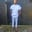Related Tags

random
elixir
random number
communitycreator

# How to generate a random number in ElixirChinweuba Elijah Azubuike

### What is a random number?

A random number is a number chosen at random from a given distribution. Selecting a large number of them reproduces the underlying distribution.

### Overview

Elixir lacks a module to produce random numbers. However, it can use underlying Erlang modules like rand (now favored over random) or crypto to select random items from enumerables.

We should note that :rand.uniform(n) returns integers. Enum.random's documentation also claims that it can effectively generate random numbers by choosing a value at random from the range limit.

This allows us to create integers inside range bounds without traversing the entire range.

### How to generate random numbers in Elixir

We use the :rand.uniform(n) method to generate random numbers in Elixir.

### Syntax

:rand.uniform(n)

### Parameters

The :rand.uniform(n) receives a number/integer n as a parameter, which represents a range of 0 to n. For example, if we want a random number between 0 and 50, we pass in n = 50.

Note: The given range is inclusive of 0 and n.

# prints a random number
random_number = :rand.uniform(256)
IO.inspect random_number
A random number between 0 and 256

### Example explained

Line 2: We create a variable random_number, where we use :rand.uniform(256). This selects a random number between 0 and 256, and passes it to the random_number variable.

Line 3: We use IO.inspect to generate the output.

### Output

Let’s run the code above to see the output as it generates a random number.

RELATED TAGS

random
elixir
random number
communitycreator

CONTRIBUTORChinweuba Elijah Azubuike
RELATED COURSES

View all Courses

Keep Exploring

Learn in-demand tech skills in half the time